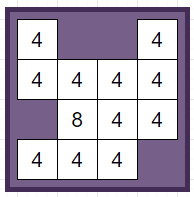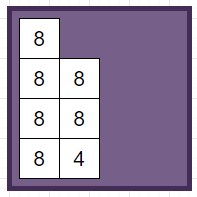# Program to find next board position after sliding the given direction once in Python

Suppose we have a 2048 game board representing the initial board and a string direction representing the swipe direction, we have to find the next board state. As we know in the 2048 game, we are given a 4 x 4 board of numbers (some of them are empty, represented in here with 0) which we can swipe in any of the 4 directions ("U", "D", "L", or "R"). When we swipe, all the numbers move in that direction as far as possible and identical adjacent numbers are added up exactly once.

So, if the input is likedirection = "L", then the output will beTo solve this, we will follow these steps:

• if direction is same as "R", then

• board := rotate board anti clockwise two times

• otherwise when direction is same as "U", then

• board := rotate board anti clockwise once

• otherwise when direction is same as "D", then

• board := rotate board anti clockwise three times

• for i in range 0 to 3, do

• row := a list of all non-zero elements at board[i]

• for j in range 0 to 2, do

• if j + 1 < size of row and row[j] is same as row[j + 1], then

• row[j] := row[j] * 2

• remove row[j + 1]

• while size of row < 4, do

• insert 0 at the end of row

• board[i] := row

• if direction is same as "R", then

• board := rotate board anti clockwise two times

• otherwise when direction is same as "U", then

• board := rotate board anti clockwise three times

• otherwise when direction is same as "D", then

• board := rotate board anti clockwise once

• return board

Let us see the following implementation to get better understanding

## Example

Live Demo

class Solution:
def solve(self, board, direction):
if direction == "R":
board = rot_anti_clock_dir(rot_anti_clock_dir(board))
elif direction == "U":
board = rot_anti_clock_dir(board)
elif direction == "D":
board = rot_anti_clock_dir(rot_anti_clock_dir(rot_anti_clock_dir(board)))

for i in range(4):
row = [x for x in board[i] if x]
for j in range(3):
if j + 1 < len(row) and row[j] == row[j + 1]:
row[j] *= 2
del row[j + 1]
while len(row) < 4:
row += 
board[i] = row

if direction == "R":
board = rot_anti_clock_dir(rot_anti_clock_dir(board))
elif direction == "U":
board = rot_anti_clock_dir(rot_anti_clock_dir(rot_anti_clock_dir(board)))
elif direction == "D":
board = rot_anti_clock_dir(board)
return board

def rot_anti_clock_dir(x):
x = [[x[i][j] for i in range(4)] for j in range(4)]
return x[::-1]

ob = Solution()
matrix = [
[2, 0, 0, 2],
[2, 2, 2, 2],
[0, 4, 2, 2],
[2, 2, 2, 0]]
print(ob.solve(matrix, "L"))

## Input

matrix = [
[2, 0, 0, 2],
[2, 2, 2, 2],
[0, 4, 2, 2],
[2, 2, 2, 0]]

## Output

[
[4, 0, 0, 0],
[4, 4, 0, 0],
[4, 4, 0, 0],
[4, 2, 0, 0]]